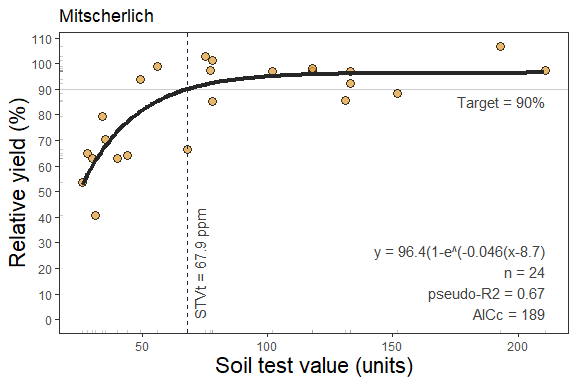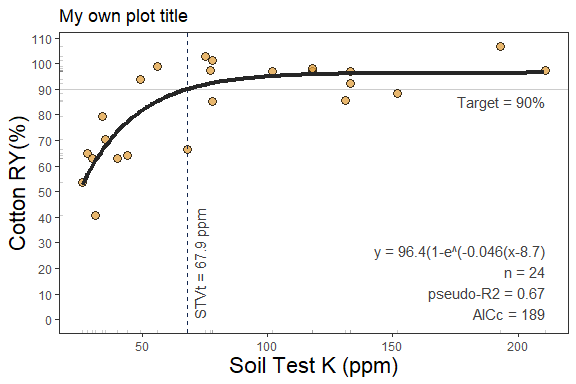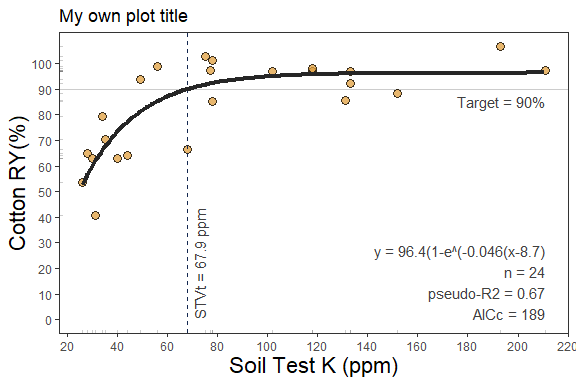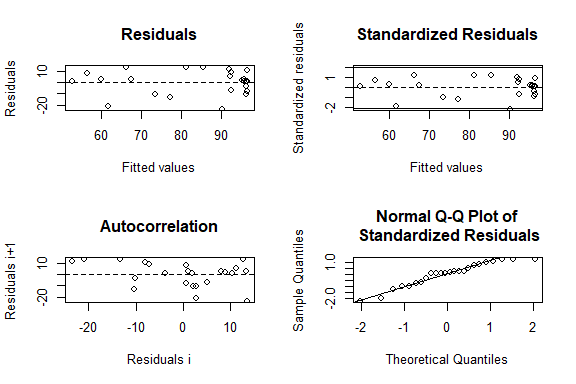# Mitscherlich-type response## Description

This tutorial demonstrates the mitscherlich() function for fitting a continuous response model and estimating a critical soil test value. This function fits a Mitscherlich-type exponential regression model that follows a diminishing growth curve described as y = a * (1-exp(-c(x + b)), where a = asymptote, b = x-intercept, c = rate or curvature parameter. This approach is extensively used in agriculture to describe crops response to input since the biological meaning of its curved response. With 3 alternatives to fit the model, the mitscherlich() function brings the advantage of controlling the parameters quantity: i) type = 1 (DEFAULT), corresponding to the model without any restrictions to the parameters (y = a * (1-exp(-c(x + b))); ii) type = 2 (“asymptote 100”), corresponding to the model with only 2 parameters by setting the asymptote = 100 (y = 100 * (1-exp(-c(x + b))), and iii) type = 3 (“asymptote 100 from 0”), corresponding to the model with only 1 parameter by constraining the asymptote = 100 and xintercept = 0 (y = 100 * (1-exp(-c(x))).

Disadvantages this model might include: i) lacks a parameter corresponding directly with a critical soil test value (the model cannot be evaluated at the asymptote as it would result in a CSTV equal to Inf); and ii) there is no apparent confidence interval for an estimated CSTV. For this latter purpose, we recommend the user would to use a re-sampling technique (e.g. bootstrapping) for a reliable confidence interval estimation of parameters and CSTV (for examples on bootstrapping, see nlraa package vignette. The mitscherlich() function works automatically with self-starting initial values to facilitate the model’s convergence.

## General Instructions

1. Load your dataframe with soil test value (stv) and relative yield (ry) data.

2. Specify the following arguments into the function -mitscherlich()-:

(a). type select the type of parameterization of the model. i) type = 1 corresponds to the model without any restrictions to the parameters (y = a * (1-exp(-c(x + b))); ii) type = 2 (“asymptote 100”), corresponding to the model with only 2 parameters by setting the asymptote = 100 (y = 100 * (1-exp(-c(x + b))), and iii) type = 3 (“asymptote 100 from 0”), corresponding to the model with only 1 parameter by constraining the asymptote = 100 and xintercept = 0 (y = 100 * (1-exp(-c(x))).

(b). data (optional),

(c). stv (soil test value) and ry (relative yield) columns or vectors,

(d). target (optional) if want to know the stv level needed for a specific ry.

(e). tidy TRUE (produces a data.frame with results) or FALSE (store results as list),

(f). plot TRUE (produces a ggplot as main output) or FALSE (no plot, only results as data.frame),

(g). resid TRUE (produces plots with residuals analysis) or FALSE (no plot),

1. Run and check results.

2. Check residuals plot, and warnings related to potential limitations of this model.

3. Adjust curve plots as desired.

# Tutorial

library(soiltestcorr)

Suggested packages

# Install if needed
library(ggplot2) # Plots
library(dplyr) # Data wrangling
library(tidyr) # Data wrangling
library(utils) # Data wrangling
library(data.table) # Mapping
library(purrr) # Mapping

This is a basic example using three different datasets:


# Example 1 dataset
# Fake dataset manually created
data_1 <- data.frame("RY"  = c(65,80,85,88,90,94,93,96,97,95,98,100,99,99,100),
"STV" = c(1,2,3,4,5,6,7,8,9,10,11,12,13,14,15))

# Example 2. Native fake dataset from soiltestcorr package

data_2 <- soiltestcorr::data_test

# Example 3. Native dataset from soiltestcorr package, Freitas et al.  (1966), used by Cate & Nelson (1971)
data_3 <- soiltestcorr::freitas1966

# Fit mitscherlich()

## 1. Individual fits

### 1.1.a type = 1

Type = “no restrictions” or Type = 1 for model with ‘no restrictions’


fit_1_type_1 <-
soiltestcorr::mitscherlich(data = data_1,
ry = RY,
stv = STV,
type = 1,
target = 90)

#> $a #>  98.71 #> #>$b
#>  2.07
#>
#> $c #>  0.37 #> #>$equation
#>  "98.7(1-e^(-0.371(x+2.1)"
#>
#> $target #>  90 #> #>$CSTV
#>  4.5

### 1.1.b type = 2

Type = “asymptote 100” or Type = 2 for model with ‘asymptote = 100’


fit_1_type_2 <-
soiltestcorr::mitscherlich(data = data_1,
ry = RY,
stv = STV,
type = 2,
target = 90)

#> $a #>  100 #> #>$b
#>  2.56
#>
#> $c #>  0.32 #> #>$equation
#>  "100(1-e^(-0.318(x+2.6)"
#>
#> $target #>  90 #> #>$CSTV
#>  4.7

### 1.1.c type = 3

Type = “asymptote 100 from 0” or Type = 3 for model with ‘asymptote = 100 and xintercept = 0’


fit_1_type_3 <-
soiltestcorr::mitscherlich(data = data_1,
ry = RY,
stv = STV,
type = 3,
target = 90)

#> $a #>  100 #> #>$b
#>  0
#>
#> $c #>  0.81 #> #>$equation
#>  "100(1-e^(-0.811x)"
#>
#> $target #>  90 #> #>$CSTV
#>  2.8

RY type = 1, target = 90%, confidence level = 0.95, replace with your desired values

### 1.2. tidy = FALSE

It returns a LIST (more efficient for multiple fits at once)


# Using dataframe argument, tidy = FALSE -> return a LIST
fit_1_tidy_false <-
soiltestcorr::mitscherlich(data = data_1,
ry = RY,
stv = STV, type = 1, target = 90,
tidy = FALSE)

#> $a #>  98.71 #> #>$b
#>  2.07
#>
#> $c #>  0.37 #> #>$equation
#>  "98.7(1-e^(-0.371(x+2.1)"
#>
#> $target #>  90 #> #>$CSTV
#>  4.5

### 1.3. tidy = TRUE

It returns a data.frame (more organized results)


# Using dataframe argument, tidy = FALSE -> return a LIST
fit_1_tidy_true <-
soiltestcorr::mitscherlich(data = data_1,
ry = RY,
stv = STV, type = 1, target = 90,
tidy = TRUE)

fit_1_tidy_true
#>       a    b    c                equation target CSTV AIC AICc   R2
#> 1 98.71 2.07 0.37 98.7(1-e^(-0.371(x+2.1)     90  4.5  64   68 0.97

### 1.4. Alternative using the vectors

You can call stv and ry vectors using the $. The tidy argument still applies for controlling the output type  fit_1_vectors_list <- soiltestcorr::mitscherlich(ry = data_1$RY,
stv = data_1$STV, type = 1, tidy = FALSE) fit_1_vectors_tidy <- soiltestcorr::mitscherlich(ry = data_1$RY,
stv = data_1$STV, type = 1, tidy = TRUE) ### 1.5. Data 2. Test dataset  fit_2 <- soiltestcorr::mitscherlich(data = data_2, ry = RY, stv = STV, type = 1, target = 90) utils::head(fit_2) #>$a
#>  97.98
#>
#> $b #>  3.91 #> #>$c
#>  0.09
#>
#> $equation #>  "98(1-e^(-0.089(x+3.9)" #> #>$target
#>  90
#>
#> $CSTV #>  24.4 ### 1.6. Data 3. Freitas et al. 1966  fit_3 <- soiltestcorr::mitscherlich(data = data_3, ry = RY, stv = STK, type = 1, target = 90) utils::head(fit_3) #>$a
#>  96.38
#>
#> $b #>  -8.69 #> #>$c
#>  0.05
#>
#> $equation #>  "96.4(1-e^(-0.046(x-8.7)" #> #>$target
#>  90
#>
stv = .$STV, type = 1, target = 90, tidy = TRUE))) utils::head(fit_multiple_map) #> # A tibble: 3 × 3 #> id data models #> <chr> <list> <list> #> 1 1 <tibble [15 × 2]> <df [1 × 9]> #> 2 2 <tibble [137 × 2]> <df [1 × 9]> #> 3 3 <tibble [24 × 2]> <df [1 × 9]> unnest(fit_multiple_map, models) #> # A tibble: 3 × 11 #> id data a b c equation target CSTV AIC AICc R2 #> <chr> <list> <dbl> <dbl> <dbl> <chr> <dbl> <dbl> <dbl> <dbl> <dbl> #> 1 1 <tibble> 98.7 2.07 0.37 98.7(1-e^(-0.… 90 4.5 64 68 0.97 #> 2 2 <tibble> 98.0 3.91 0.09 98(1-e^(-0.08… 90 24.4 1022 1022 0.54 #> 3 3 <tibble> 96.4 -8.69 0.05 96.4(1-e^(-0.… 90 67.9 187 189 0.67 ### 2.1. Using group_map Alternatively, with group_map, we do not require nested data. However, it requires to dplyr::bind_rows and add an id column specifying the name of each dataset. This option return models as lists objects.  fit_multiple_group_map <- dplyr::bind_rows(data_1, data_2, .id = "id") %>% dplyr::group_by(id) %>% dplyr::group_map(~ soiltestcorr::mitscherlich(data = ., ry = RY, stv = STV, type = 1, target = 90, tidy = TRUE)) utils::head(fit_multiple_group_map) #> [] #> a b c equation target CSTV AIC AICc R2 #> 1 98.71 2.07 0.37 98.7(1-e^(-0.371(x+2.1) 90 4.5 64 68 0.97 #> #> [] #> a b c equation target CSTV AIC AICc R2 #> 1 97.98 3.91 0.09 98(1-e^(-0.089(x+3.9) 90 24.4 1022 1022 0.54 ## 3. Plots ### 3.1. Calibration Curve We can generate a ggplot with the same mitscherlich() function. We just need to specify the argument plot = TRUE.  mitscherlich_plot <- soiltestcorr::mitscherlich(data = data_3, ry = RY, stv = STK, type = 1, target = 90, plot = TRUE) mitscherlich_plot### 3.1.2 Fine-tune the plots As ggplot object, plots can be adjusted in several ways. For example, modifying titles mitscherlich_plot_2 <- mitscherlich_plot + labs( # Main title title = "My own plot title", # Axis titles x = "Soil Test K (ppm)", y = "Cotton RY(%)") mitscherlich_plot_2Or modifying axis scales mitscherlich_plot_3 <- mitscherlich_plot_2 + # Axis scales scale_x_continuous(breaks = seq(0,220, by = 20))+ # Axis limits scale_y_continuous(breaks = seq(0,100, by = 10)) mitscherlich_plot_3### 3.3. Residuals We can generate a plot with the same mitscherlich() function. We just need to specify the argument resid = TRUE.  # Residuals plot soiltestcorr::mitscherlich(data = data_3, ry = RY, stv = STK, type = 1, target = 90, resid = TRUE)#>$a
#>  96.38
#>
#> $b #>  -8.69 #> #>$c
#>  0.05
#>
#> $equation #>  "96.4(1-e^(-0.046(x-8.7)" #> #>$target
#>  90
#>
#> $CSTV #>  67.9 #> #>$AIC
#>  187
#>
#> $AICc #>  189 #> #>$R2
#>  0.67`

References

Melsted, S.W. and Peck, T.R. (1977). The Mitscherlich-Bray Growth Function. In Soil Testing (eds T. Peck, J. Cope and D. Whitney). 10.2134/asaspecpub29.c1